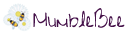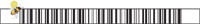# Free Amazon Android App of the Day 5/28/2014Click image to enter!

Free Amazon Android App of the day for 5/28/2014 only!

Normally \$5.99 but for today it is FREE!!

Graphing calculator with algebra. Essential tool for school and college. Replaces bulky and expensive handheld graphing calculators.

Multiple functions on a graph, polar graphs, graphing of implicit functions, values and slopes, roots, extremes, intersections. Algebra: polynomials, polynomial equation solving, matrices, fractions, derivatives, complex numbers and more. Shows results as you type. Use menu to switch between modes.

Help site with instructions and examples: http://help.mathlab.us
If you have a question, send email to calc@mathlab.us

§ SCIENTIFIC CALCULATOR
• arithmetic expressions +,-,*,/,÷
• square root, cube and higher roots (hold root key)
• exponent, logarithms (ln, log)
• trigonometric functions sin π/2, cos 30°, …
• hyperbolic functions sinh, cosh, tanh, … (hold “e” key to switch)
• inverse functions (hold direct function key)
• complex numbers, all functions support complex arguments
• derivatives sin x’ = cos x, … (hold x^n key)
• scientific notation (enable in menu)
• percent mode

§ GRAPHING CALCULATOR
• multiple functions graphing
• implicit functions up to 2nd degree (ellipse 2x^2+3y^2=1, etc.)
• polar graphs (r=cos2θ)
• parametric functions, enter each on new line (x=cos t, y=sin t)
• function roots and critical points on a graph, tap legend to turn on and off (top left corner), use menu to display as a list
• graph intersections (x^2=x+1)
• tracing function values and slopes
• scrollable and resizable graphs
• pinch to zoom
• fullscreen graphs in landscape orientation
• function tables
• save graphs as images
• save tables as csv

§ FRACTION CALCULATOR
• simple and complex fractions 1/2 + 1/3 = 5/6
• mixed numbers, use space to enter values 3 1/2

§ ALGEBRA CALCULATOR
• linear equations x+1=2 -> x=1
• quadratic equations x^2-1=0 -> x=-1,1
• approximate roots of higher polynomials
• systems of linear equations, write one equation per line, x1+x2=1, x1-x2=2
• polynomial long division
• polynomial expansion, factoring

§ MATRIX CALCULATOR
• matrix and vector operations
• dot product (hold *), cross product
• determinant, inverse, norm, transpose, trace

§ LIBRARY
• user defined constants and functions (PRO)
Follow Me On Social Media